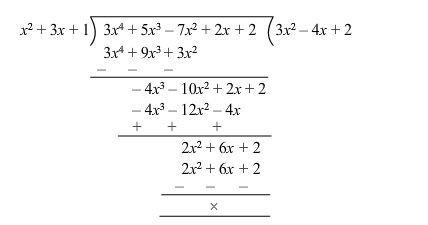# Find the quotient when p(x)Question:

Find the quotient when $p(x)=3 x^{4}+5 x^{3}-7 x^{2}+2 x+2$ is divided by $\left(x^{2}+3 x+1\right)$.

Solution:

Given : $p(x)=3 x^{4}+5 x^{3}-7 x^{2}+2 x+2$

Dividing $p(x)$ by $\left(x^{2}+3 x+1\right)$, we have :$\therefore$ The quotient is $3 x^{2}-4 x+2$